Vol. Practical Guide to Radio-Frequency Analysis and Design

# Phase Modulation: Theory, Time Domain, Frequency Domain

## Phase modulation is similar to frequency modulation and is an important technique in digital communication systems.

Phase modulation is similar to frequency modulation and is an important technique in digital communication systems.

We have all heard of AM radio and FM radio. But phase modulation seems to be in a different category—“PM radio” is by no means a common term. It turns out that phase modulation is more relevant in the context of digital RF. In a way, though, we can say that PM radio is as common as FM radio simply because there is little difference between phase modulation and frequency modulation. FM and PM are best considered as two closely related variants of angle modulation, where “angle” refers to the modification of the quantity passed to a sine or cosine function.

### The Math

We saw in the previous page that frequency modulation is achieved by adding the integral of the baseband signal to the argument of a sine or cosine function (where the sine or cosine function represents the carrier):

$$x_{FM}(t)=\sin(\omega_Ct+\int_{-\infty}^{t} x_{BB}(t)dt)$$

You will recall, though, that we introduced frequency modulation by first discussing phase modulation: adding the baseband signal itself, rather than the integral of the baseband signal, causes the phase to vary according to the baseband value. Thus, phase modulation is actually a bit simpler than frequency modulation.

$$x_{PM}(t)=\sin(\omega_Ct+x_{BB}(t))$$

As with frequency modulation, we can use the modulation index to make the phase variations more sensitive to the changes in the baseband value:

$$x_{PM}(t)=\sin(\omega_Ct+mx_{BB}(t))$$

The similarity between phase modulation and frequency modulation becomes clear if we consider a single-frequency baseband signal. Let’s say that xBB(t) = sin(ωBBt). The integral of sine is negative cosine (plus a constant, which we can ignore here)—in other words, the integral is simply a time-shifted version of the original signal. Thus, if we perform phase modulation and frequency modulation with this baseband signal, the only difference in the modulated waveforms will be the alignment between the baseband value and the variations in the carrier; the variations themselves are the same. This will be more clear in the next section, where we’ll look at some time-domain plots.

It’s important to keep in mind that we’re dealing with instantaneous phase, just as frequency modulation is based on the concept of instantaneous frequency. The term “phase” is rather vague. One familiar meaning refers to the initial state of a sinusoid; for example, a “normal” sine wave begins with a value of zero and then increases toward its maximum value. A sine wave that begins at a different point in its cycle has a phase offset. We can also think of phase as a specific portion of a full waveform cycle; for example, at a phase of π/2, a sinusoid has completed one-fourth of its cycle.

These interpretations of “phase” don’t help us very much when we’re dealing with a phase that continuously varies in response to a baseband waveform. Rather, we use the concept of instantaneous phase, i.e., the phase at a given moment, which corresponds to the value passed (at a given moment) to a trigonometric function. We can think of these continuous variations in instantaneous phase as “pushing” the carrier value farther from or closer to the preceding state of the waveform.

One more thing to keep in mind: Trig functions, including sine and cosine, operate on angles. Changing the argument of a trig function is equivalent to changing the angle, and this explains why both FM and PM are described as angle modulation.

### The Time Domain

We’ll use the same waveforms that we used for the FM discussion, i.e., a 10 MHz carrier and a 1 MHz sinusoidal baseband signal: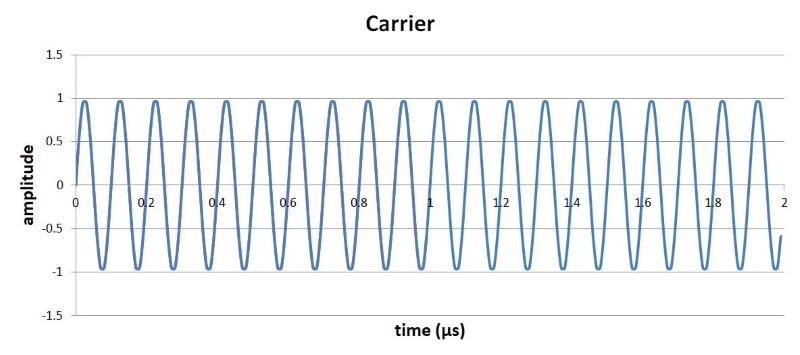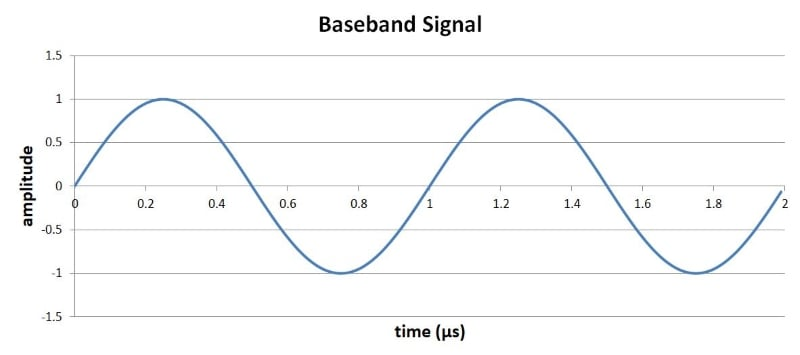Here is the FM waveform (with m = 4) that we saw in the previous page: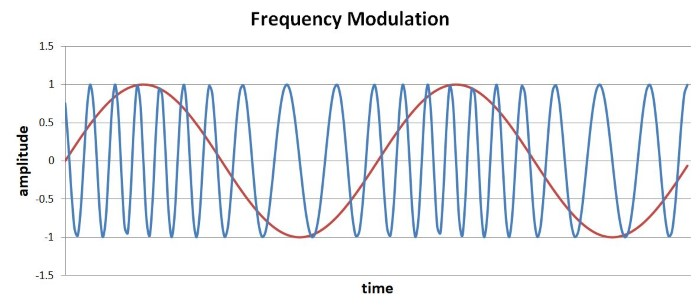We can compute the PM waveform by using the following equation, where the signal added to the argument of the carrier wave uses positive sine (i.e., the original signal) instead of negative cosine (i.e., the integral of the original signal).

$$x_{PM}(t)=\sin((10\times10^6\times2\pi t)+\sin(1\times10^6\times2\pi t))$$

Here is the PM plot: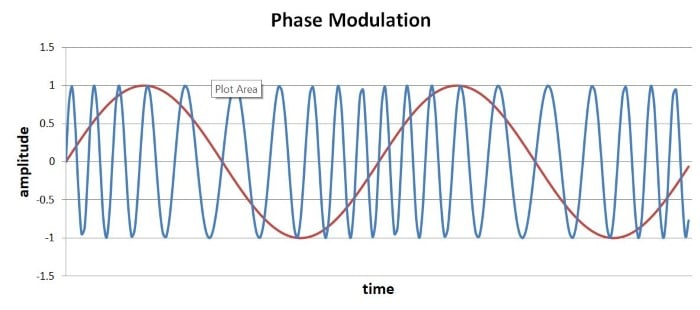Before we discuss this, let’s look also at a plot that shows the FM waveform and the PM waveform: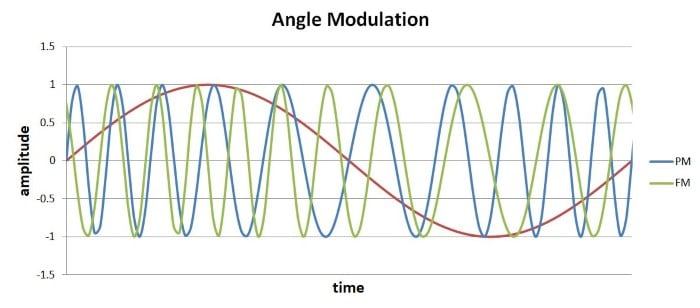The first thing that comes to mind here is that from a visual standpoint, FM is more intuitive than PM—there is a clear visual connection between the higher- and lower-frequency sections of the modulated waveform and the higher and lower baseband values. With PM, the relationship between the baseband waveform and the behavior of the carrier is perhaps not immediately apparent. However, after a bit of inspection we can see that the PM carrier frequency corresponds to the slope of the baseband waveform; the highest-frequency sections occur during the steepest positive slope of xBB, and the lowest-frequency sections occur during the steepest negative slope.

This makes sense: Recall that frequency (as a function of time) is the derivative of phase (as a function of time). With phase modulation, the slope of the baseband signal governs how quickly the phase changes, and the rate at which the phase changes is equivalent to frequency. So in a PM waveform, high baseband slope corresponds to high frequency, and low baseband slope corresponds to low frequency. With frequency modulation, we use the integral of xBB, which has the effect of shifting the high- (or low-) frequency carrier sections to the baseband values following the high- (or low-) slope portions of the baseband waveform.

### The Frequency Domain

The preceding time-domain plots demonstrate what was said previously: frequency modulation and phase modulation are quite similar. It is not surprising, then, that PM’s effect in the frequency domain is similar to that of FM. Here are spectra for phase modulation with the carrier and baseband signals used above: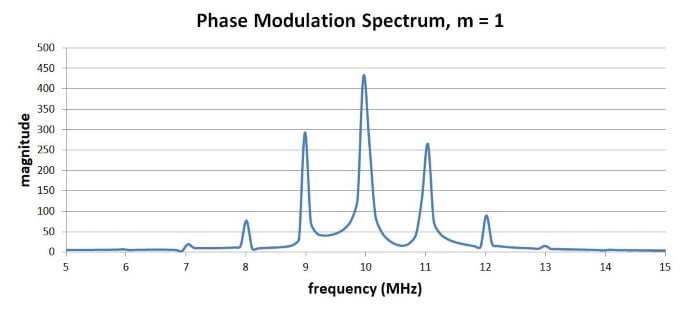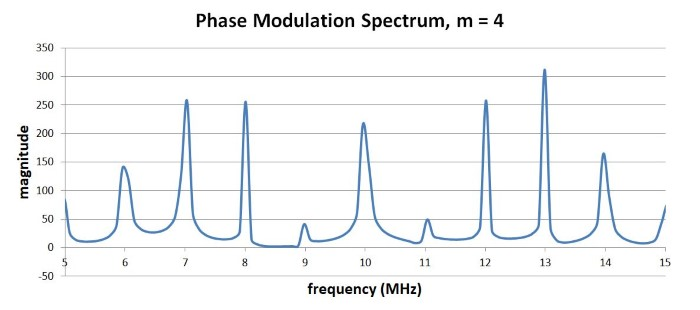### Summary

• Phase modulation is calculated by adding the baseband signal to the argument of a sine or cosine function that represents the carrier.
• The modulation index makes the phase variations more or less sensitive to the behavior of the baseband signal.
• The frequency-domain effects of phase modulation are similar to those of frequency modulation.
• Analog phase modulation is not common; however, digital phase modulation is widely used.

#### Lessons in Electric Circuits

Published under the terms and conditions of the Design Science License